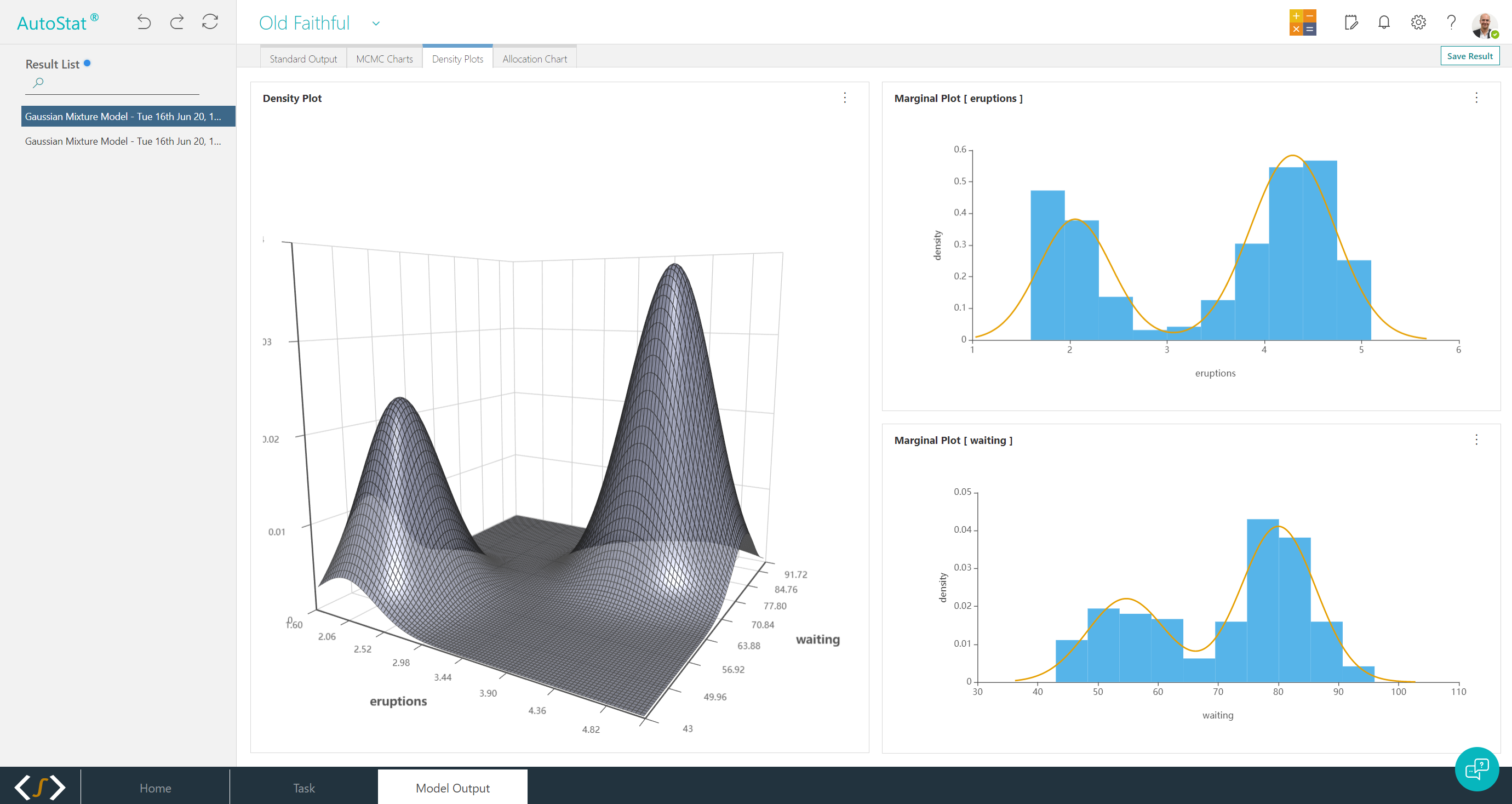## Model Builder

AutoStat®’s engine room. Undertake a range of advanced analytics with your data and apply machine learning, statistics, time-series and survival modelling without needing to code. Bayesian optimisation with AutoML for automated variable selection and parameter tuning to enhance model performance.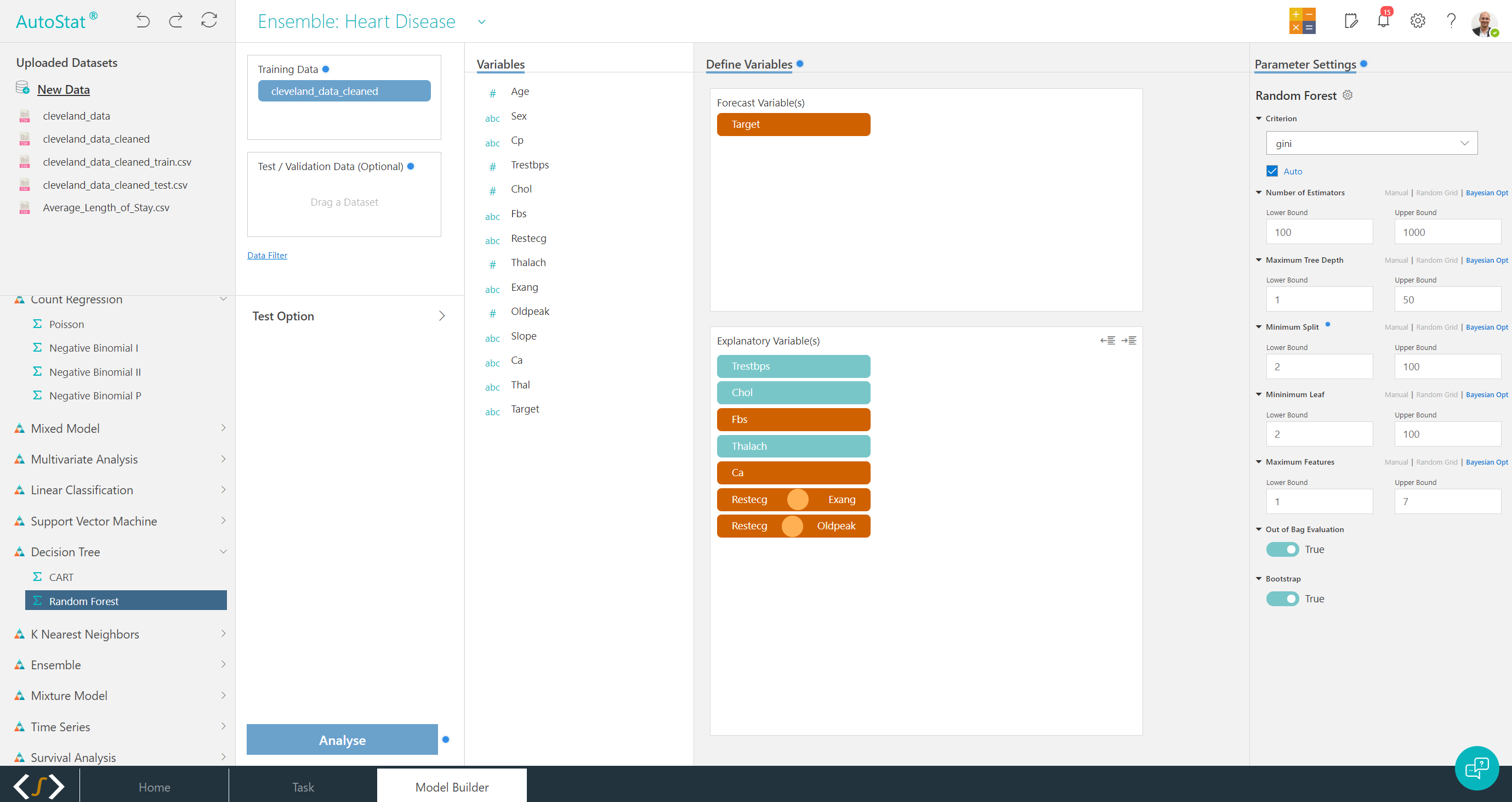## 01 - Statistical FrameworksModel a wide range of data using linear regression & mixed models, along with a range of generalised linear models, including Logistic, Probit, Poisson, Logit and Tobit, plus extensions for mixed effects models.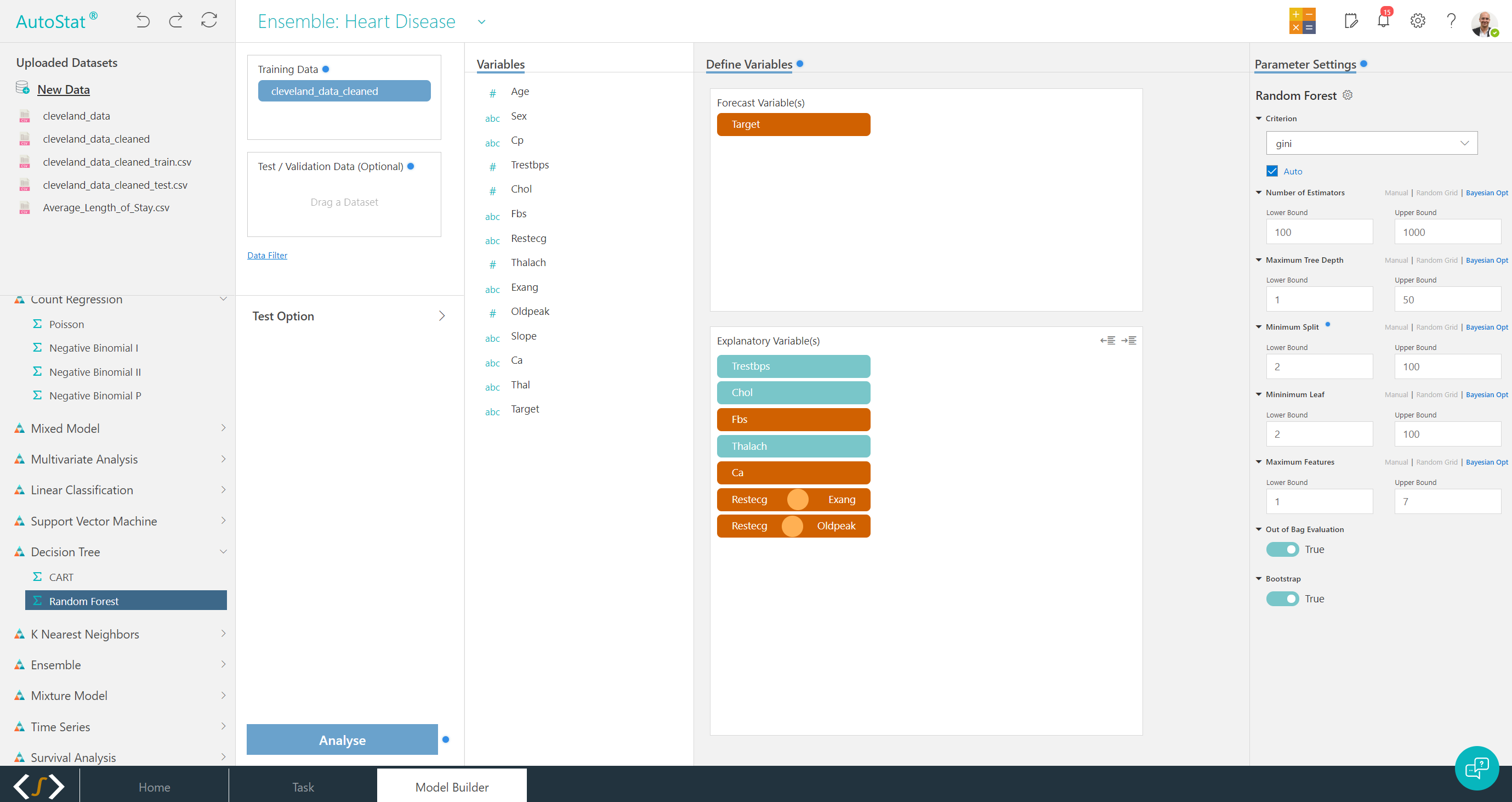## 02 - Machine Learning and ClusteringAccess a range of supervised and unsupervised machine learning algorithms for both regression and classification problems. Apply clustering with Gaussian mixture models and a number of ML clustering algorithms.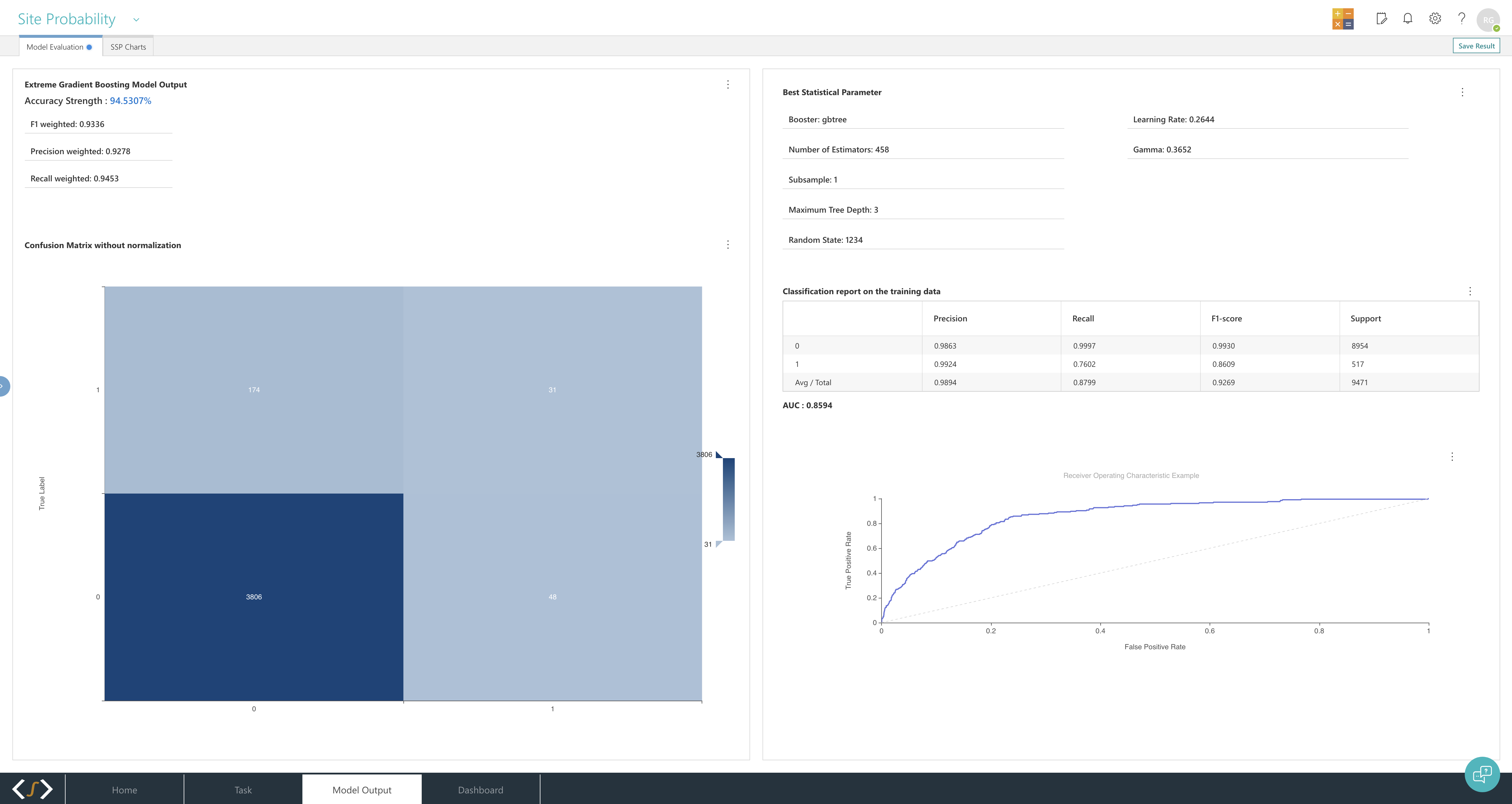## 03 - Multivariate ModellingMake prediction models across many combinations of variables. Including dynamic factor models, factor analysis, multiple regression and linear discriminant analysis.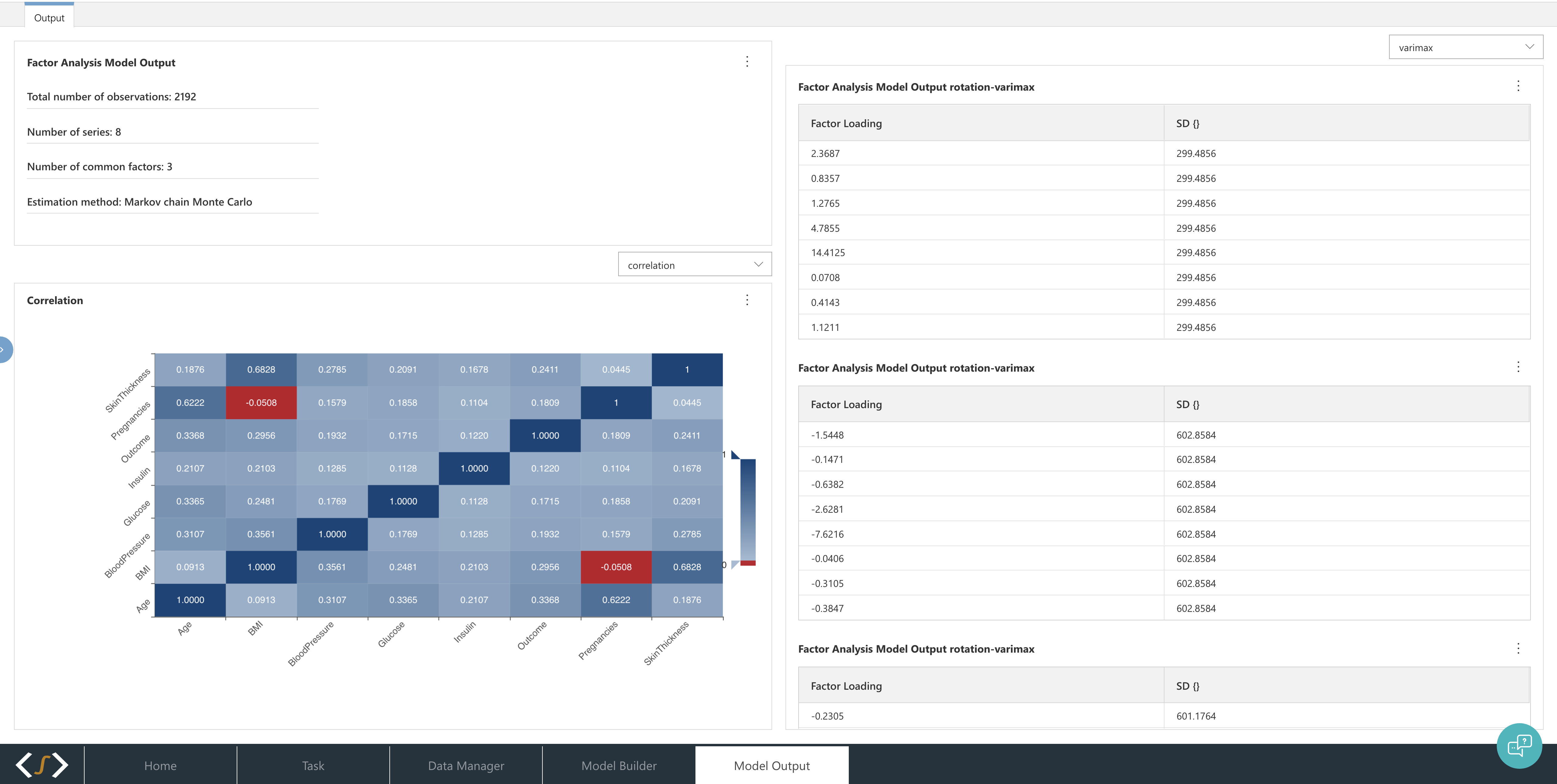## 04 - Time-Series ModellingExtract forecasts and identify trends with some of the most advanced time-series forecasting models including ARIMA, S-ARIMA, and Bayesian Unobserved Component models.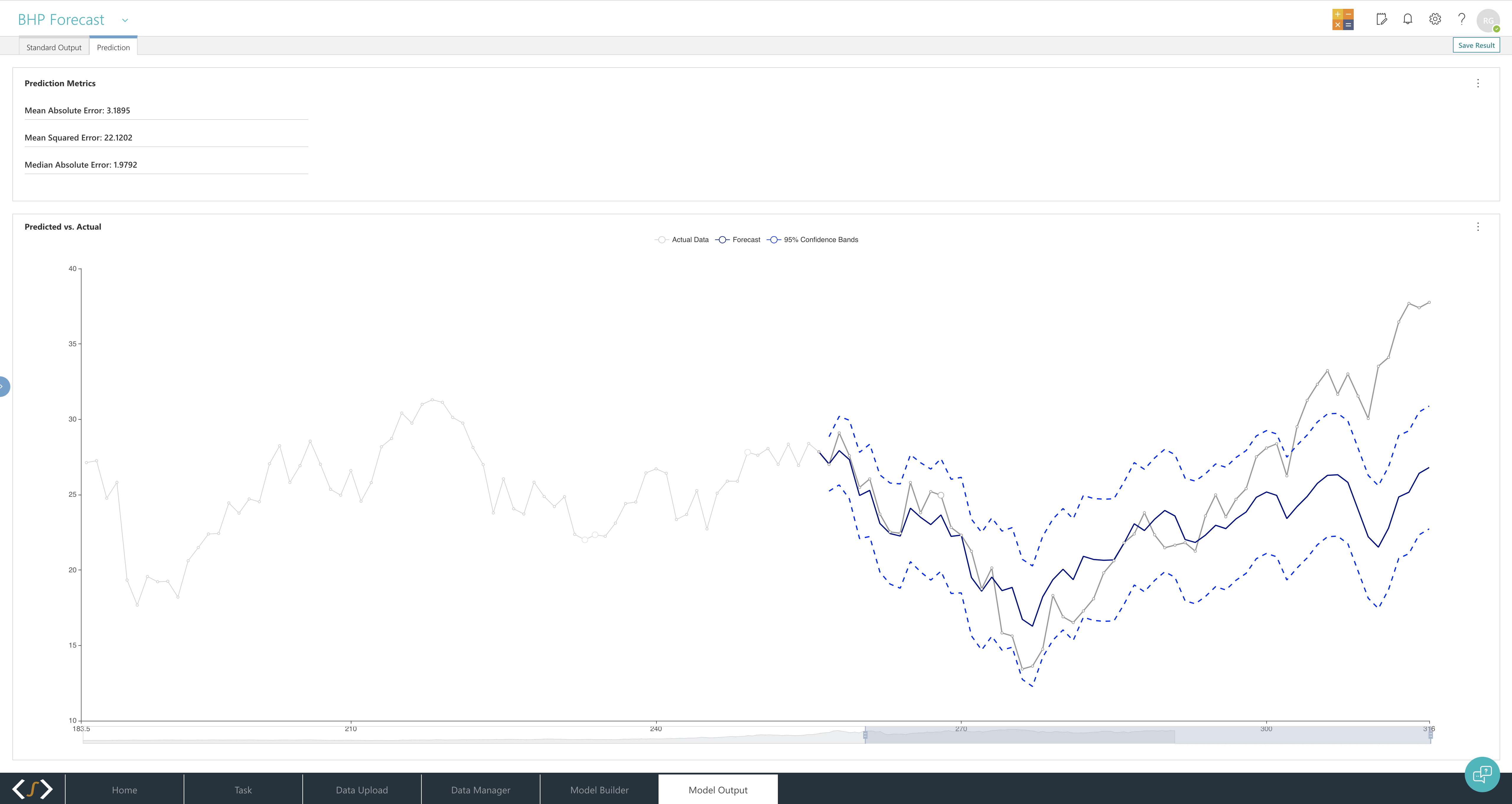## 05 - Survival AnalysisAnalyse failure points and durations of variables using a range of parametric and non-parametric algorithms.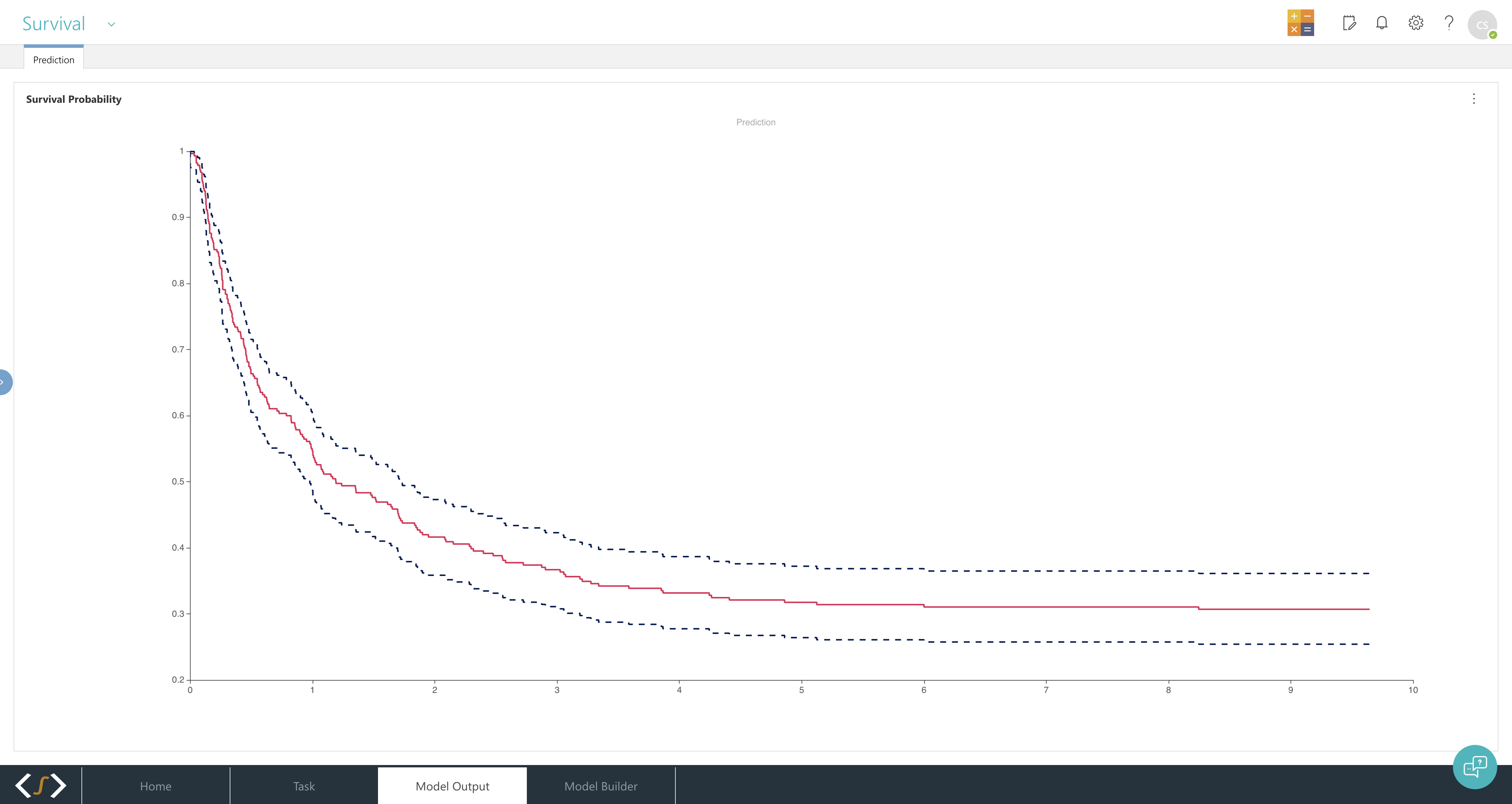## 06 - Results AnalyticsAnalyse model performance, identify key variables and their relative importances, and extract predictions for real-world insights.